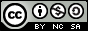- 目录 -
LeetCode 1248 统计「优美子数组」(前缀和，思路)

输入：nums = [1,1,2,1,1], k = 3



输入：nums = [2,4,6], k = 1



输入：nums = [2,2,2,1,2,2,1,2,2,2], k = 2



• 1 <= nums.length <= 50000
• 1 <= nums[i] <= 10^5
• 1 <= k <= nums.length

## 代码

  1 2 3 4 5 6 7 8 9 10 11 12 13 14 15 16 17  class Solution { public: int numberOfSubarrays(vector &nums, int k) { unordered_map mp; mp = 1; int sum = 0, ans = 0; for (int i = 1; i <= nums.size(); i++) { sum += nums[i - 1] & 1; ans += mp[sum - k]; mp[sum]++; } return ans; } };